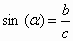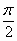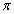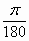# Translations of Encyclopedia about Mathematics

## Trigonometric Functions and their Procedures

Trigonometric functions describe the relation between the sides and angles of a triangle and can be used to make calculations with unitary circles. Unitary circles are circles which have a diameter of 1 unit and whose centre passes through a co-ordinal system. The unit of length can be anything.

If we let the positive part of an axis x rotate around a centre, we get an angle a . If a point P with angle a lies on the unitary ring, its coordinate x relates to the cosine of angle a and its coordinate y of the sine of angle a .

For setting values, a right angle triangle is used, one of whose corners lies at the origin of the coordinate system. A hypotenuse is a one unit long unit. The starting point of a hypotenuse is the origin of the coordinate system and its end lies on the unitary ring. For each imaginary point in the circle, one can draw a triangle which is bordered by this point in the circle, the origin of the coordinate system, and some point on axis x. The point on axis x is chosen to make the triangle a right angle triangle.

The tangent lies opposite the right angle and the adjoining leg lies on the decisive angle, across from which lies the opposite leg.

Now we can draw graphs of these trigonometric functions, as with other functions. It will not come out of the actual lengths of the absissae but, for example, with the opposite leg, from the value of coordinate Y and, with the adjoining leg, from the value x. Depending on where we are located, we can also attain a negative value. Furthermore, the angle attained can be greater than 360° - for example, we can attain an angle of 400 from a value of 40 (400-360), although our end result is the same.

The sine (cosine) of negative angles -a equals the sine (cosine) of the angle (360 - a ).

If we imagine a unitary circle as a clock dial and that the axis x circumscribes one rotation along the rotation of a watch hand, for example by an amount of 45°, then the rotation of axis x in the opposite direction of the rotation of the watch hand would have a negative sign, for example -45°. The sine of -45° is equal to the sine of (360° - 45° )

 The sine (cosine) of angle (n.360° + a ), where n Î IN, equals the sine (cosine) of angle a .

An angle of 60° corresponds to an angle of 420°, which has the same position in a coordinate system only that it has made one rotation more.

Expanding the Definition of Sine in a Right Angle Triangle and the Definition of a Unitary Circle

The definition of the sine in a right angle triangle is as follows: sine a = the length of the leg lying opposite the hypotenuse.The hypotenuse has a value of one, while it applies that:

sin a = the length of the opposite leg.

The opposite leg equals the coordinate y of point P and may be written as:

sin a = coordinate y of point P.

This is also the definition of sine using a unitary circle. This means that both definitions are identical in the range 0° < a < 90°. However, in a unitary ring, the value of sine can be assigned to angles larger than 90° (contrary to right angle triangles). For each angle a in a unitary ring, there exists a defined value for sine and cosine. Each value of sine can be assigned some angle a , although this assignment is not definite.

Sine

If we have an angle a = 45°, we determine the length of the opposing leg to give us a value of 0.7.

This value is carried over to the coordinate system, whose axis y attains a value between -1 and +1 and whose axis x corresponds to the degrees. We therefore carry over the point P for y = 0.7 and x = 45°. For an angle of a = 90°, we get a value of 1 (x = 90°, y = 1).

for x = 135° we get y = 0.7
for x = 180° we get y = 0
for x = 225° we get y = -0.7
for x = 270° we get y = -1

A curve is therefore made up of an arc lying in a positive area which then continues into a negative area. Sinusoids have a periodic character and repeat every 360°. It also always applies that sin a = sin(n.360° +a ) for all nÎ Z.

The sine function is indirect, as is evident from its graph which shows a point symmetry relating to the origin point of the coordinate system (relating to zero). For example, if we have a graph which starts in negative numbers, we can take a point of x = -135° and reflect rotate it around the centre of the coordinate system to give us a sine value relating to x = +135°.

Cosine

Using a unitary ring, the definition of cosine can assign an exact value corresponding to the cosine of each angle. This definite assignment is referred to as the cosine function.

cosa : the cosine of angle a equals the ratio of the length of the adjoining leg to the hypotenuse.

Following are shown some coordinates:

x = 45°, y = 0,7 x = 90°, y = 0 x = 135°, y = -0,7 x = 180°, y = -1
x = 225°, y = -0,7 x = 270°, y = 0 x = 315°, y = 0,7

The cosine function is also a periodic function which repeats itself every 360°. This means that the cosine function has a periodicity of 360°. The cosine function is a direct function and its graph is axially symmetrical to the y axis. A curve looks exactly like a sinusoid but shifted to the left.

Both functions, sine and cosine, look like waves. A curve shown by an oscilloscope when measuring electrical current looks the same - it is also a sinusoid.

Angle Measurement

In higher mathematics, an angle is measured in angle measurements. The functions of sine and cosine are closely related to complex exponential functions.

The angle of 360° relates to the arc of 2p . An angle in degree x° corresponds to the arc:The arc 2p has the length of a circle which has a diameter equal to 1 rad.

an angle of 90° corresponds to an angle ofan angle of 180° corresponds to an angle of1° can therefore be expressed asTranslating Dutch Hungarian Translations Hungarian Dutch Translating Swedish Czech Translations Czech Swedish Translating RussianEnter your search terms Submit search form